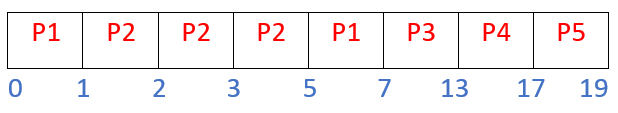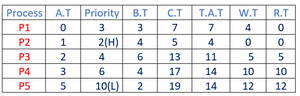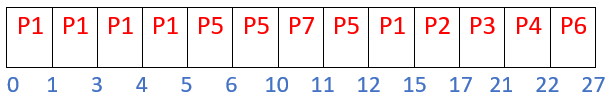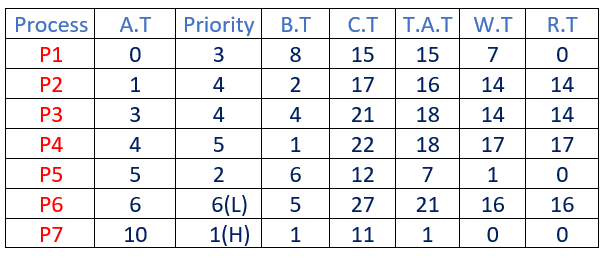Open in App
Not now

# Preemptive Priority CPU Scheduling Algorithm

• Difficulty Level : Basic
• Last Updated : 28 Dec, 2022

Preemptive Priority CPU Scheduling Algorithm is a pre-emptive method of CPU scheduling algorithm that works based on the priority of a process. In this algorithm, the scheduler schedules the tasks to work as per the priority, which means that a higher priority process should be executed first. In case of any conflict, i.e., when there is more than one process with equal priorities, then the pre-emptive priority CPU scheduling algorithm works on the basis of FCFS (First Come First Serve) algorithm.

### How does Preemptive Priority CPU Scheduling Algorithm decide the Priority of a Process?

Preemptive Priority CPU Scheduling Algorithm uses a rank-based system to define a rank for each process, where lower rank processes have higher priority and higher rank processes have lower priority. For instance, if there are 10 processes to be executed using this Preemptive Algorithm, then process with rank 1 will have the highest priority, the process with rank 2 will have comparatively lesser priority, and process with rank 10 will have least priority.

### How does Preemptive Priority CPU Scheduling Algorithm work?

• Step-1: Select the first process whose arrival time will be 0, we need to select that process because that process is only executing at time t=0.
• Step-2: Check the priority of the next available process. Here we need to check for 3 conditions.
• if priority(current_process) > priority(prior_process) :- then execute the current process.
• if priority(current_process) < priority(prior_process) :- then execute the prior process.
• if priority(current_process) = priority(prior_process) :- then execute the process which arrives first i.e., arrival time should be first.
• Step-3: Repeat Step-2 until it reaches the final process.
• Step-4: When it reaches the final process, choose the process which is having the highest priority & execute it. Repeat the same step until all processes complete their execution.

### Program for Preemptive Priority CPU Scheduling

The preemptive Priority algorithm can be implemented using Min Heap data structure. For the detailed implementation of the Preemptive priority scheduling algorithm, please refer: Program for Preemptive Priority CPU Scheduling

### Examples to show the working of Preemptive Priority CPU Scheduling Algorithm

Example-1: Consider the following table of arrival time, Priority, and burst time for five processes P1, P2, P3, P4, and P5

The Preemptive Priority CPU Scheduling Algorithm will work on the basis of the steps mentioned below:

• At time t = 0,
• Process P1 is the only process available in the ready queue, as its arrival time is 0ms.
• Hence Process P1 is executed first for 1ms, from 0ms to 1ms, irrespective of its priority.
• Remaining Burst time (B.T) for P1 = 3-1 = 2 ms.
• At time t = 1 ms,
• There are 2 processes available in the ready queue: P1 and P2.
• Since the priority of process P2 is higher than the priority of process P1, therefore Process P2 will get executed first.
• Hence Process P2 is executed for 1ms, from 1ms to 2ms.
• Remaining Burst time (B.T) for P2 = 4-1 = 3 ms.
• At time t = 2 ms,
• There are 3 processes available in the ready queue: P1, P2, and P3.
• Since the priority of process P2 is higher than the priority of process P1 and P3, therefore Process P2 will get executed first.
• Hence Process P2 is executed for 1ms, from 2ms to 3ms.
• Remaining Burst time (B.T) for P2 = 3-1 = 2 ms.
• At time t = 3 ms,
• There are 4 processes available in the ready queue: P1, P2, P3, and P4.
• Since the priority of process P2 is highest among the priority of processes P1, P2, P3, and P4, therefore Process P2 will get executed first.
• Hence Process P2 is executed for 1ms, from 3ms to 4ms.
• Remaining Burst time (B.T) for P2 = 1-1 = 0 ms.
• At time t = 4 ms,
• There are 5 processes available in the ready queue: P1, P2, P3, P4, and P5.
• Since the priority of process P2 is highest among the priority of processes P1, P2, P3, P4, and P5, therefore Process P2 will get executed first.
• Hence Process P2 is executed for 1ms, from 4ms to 5ms.
• Remaining Burst time (B.T) for P2 = 1-1 = 0 ms.
• Since Process P2’s burst time has become 0, therefore it is complete and will be removed from the process queue.
• At time t = 5 ms,
• There are 4 processes available in the ready queue: P1, P3, P4, and P5.
• Since the priority of process P1 is the highest among the priority of processes P1, P3, P4 and P5, therefore Process P1 will get executed first.
• Hence Process P1 is executed for 2ms, from 5ms to 7ms.
• Remaining Burst time (B.T) for P1 = 2-2 = 0 ms.
• Since Process P1’s burst time has become 0, therefore it is complete and will be removed from the process queue.
• At time t = 7 ms,
• There are 3 processes available in the ready queue: P3, P4, and P5.
• Since the priority of process P3 is the highest among the priority of processes P3, P4, and P5, therefore Process P3 will get executed first.
• Hence Process P3 is executed for 6ms, from 7ms to 13ms.
• Remaining Burst time (B.T) for P3 = 6-6 = 0 ms.
• Since Process P3’s burst time has become 0, therefore it is complete and will be removed from the process queue.
• At time t = 13 ms,
• There are 2 processes available in the ready queue: P4 and P5.
• Since the priority of process P4 is highest among the priority of process P4 and P5, therefore Process P4 will get executed first.
• Hence Process P4 is executed for 4ms, from 13ms to 17ms.
• Remaining Burst time (B.T) for P4 = 4-4 = 0 ms.
• Since Process P4’s burst time has become 0, therefore it is complete and will be removed from the process queue.
• At time t = 17 ms,
• There is only 1 process available in the ready queue: P5.
• Hence Process P5 is executed for 2ms, from 17ms to 19ms.
• Remaining Burst time (B.T) for P5 = 2-2 = 0 ms.
• Since Process P5’s burst time has become 0, therefore it is complete and will be removed from the process queue.
• At time t = 19 ms,
• There is no more process available in the ready queue. Hence the processing will now stop.
• The overall execution of the processes will be as shown below:

Gantt chart for above execution:Now we need to calculate the completion time (C.T) & First Arrival Time (F.A.T) of each process through Gantt chart, as per the below relations:

Turn Around Time (T.A.T) = (Completion Time) – (Arrival Time)

Waiting Time (W.T) = (Turn Around Time) – (Burst Time)

Response Time (R.T) = (First Arrival Time) – (Arrival Time)

After calculating the above fields, the final table looks likeThe final table

Here, H – Highest Priority, and L – Least Priority

Some other important relations for this example include:

• Total Turn Around Time = 7 + 4 + 11 + 14 + 14 = 50 ms
• Average Turn Around Time = (Total Turn Around Time)/(no. of processes) = 50/5 = 10.00 ms
• Total Waiting Time = 4 + 0 + 5 + 10 + 12 = 31 ms
• Average Waiting Time = (Total Waiting Time)/(no. of processes) = 31/5 = 6.20 ms
• Total Response Time = 0 + 0 + 5 + 10 + 12 = 27 ms
• Average Response Time = (Total Response Time)/(no. of processes) = 27/5 = 5.40 ms

Example 2:

Consider the following table of arrival time, Priority and burst time for seven processes P1, P2, P3, P4, P5, P6 and P7

• At time t = 0
• Process P1 is available in the ready queue, executing P1 for 1 ms
• Remaining B.T for P1 = 8-1 = 7 ms.

• At time t = 1
• The priority of P1 is greater than P2, so we execute P1 for 2 ms, from 1 ms to 3 ms.
• Remaining B.T for P1 = 7-2 = 5 ms.
• At time t = 3
• The priority of P1 is greater than P3, so we execute P1 for 1 ms.
• Remaining B.T for P1 = 5-1 = 4 ms.
• At time t = 4
• The priority of P1 is greater than P4, so we execute P1 for 1 ms.
• Remaining B.T for P1 = 4-1 = 3 ms.
• At time t = 5
• The priority of P5 is greater than P1, so we execute P5 for 1 ms.
• Remaining B.T for P5 = 6-1 = 5 ms.
• At time t = 6
• The priority of P5 is greater than P6, so we execute P5 for 4 ms.
• Remaining B.T for P5 = 5-4 = 1 ms.
• At time t = 10
• The priority of P7 is greater than P5, so we execute P7 for 1 ms.
• Remaining B.T for P7 = 1-1 = 0 ms.
• Here Process P7 completes its execution.
• At time t = 11,
• Now we take the process which is having the highest priority.
• Here we find P5 is having the highest priority & execute P5 completely
• Remaining B.T of P5 = 1-1 = 0 ms.
• Here Process P5 completes its execution.
• At time t = 12,
• Now we take the process which is having the highest priority.
• Here we find P1 is having the highest priority & execute P1 completely
• Remaining B.T of P1 = 3-3 = 0 ms.
• Here Process P1 completes its execution.
• At time t = 15,
• Now we take the process which is having the highest priority.
• Here we find P2 is having the highest priority & execute P2 completely
• Remaining B.T of P2 = 2-2 = 0 ms.
• Here Process P2 completes its execution.
• At time t = 17,
• Now we take the process which is having the highest priority.
• Here we find P3 is having the highest priority & execute P3 completely
• Remaining B.T of P3 = 4-4 = 0 ms.
• Here Process P3 completes its execution.
• At time t = 21,
• Now we take the process which is having the highest priority.
• Here we find P4 is having the highest priority & execute P4 completely
• Remaining B.T of P4 = 1-1 = 0 ms.
• Here Process P4 completes its execution.
• At time t = 22,
• Now we take the process which is having the highest priority.
• Here we find P6 is having the highest priority & execute P6 completely
• Remaining B.T of P6 = 5-5 = 0 ms.
• Here Process P6 completes its execution.

Gantt chart:Here, H – Higher Priority, L – Least Priority

### Drawbacks of Preemptive Priority Scheduling Algorithm:

One of the most common drawbacks of the Preemptive priority CPU scheduling algorithm is the Starvation Problem. This is the problem in which a process has to wait for a longer amount of time to get scheduled into the CPU. This condition is called the starvation problem.

Example: In Example 2, we can see that process P1 is having Priority 3 and we have pre-empted the process P1 and allocated the CPU to P5. Here we are only having 7 processes.
Now, if suppose we have many processes whose priority is higher than P1, then P1 needs to wait for a longer time for the other process to be pre-empted and scheduled by the CPU. This condition is called a starvation problem.

Solution: The solution to this Starvation problem is Ageing. This can be done by decrementing the priority number by a certain number of a particular process which is waiting for a longer period of time after a certain interval.

After every 3 units of time, all the processes which are in waiting for the state, the priority of those processes will be decreased by 2, So, if there is a process P1 which is having priority 5, after waiting for 3 units of time its priority will be decreased from 5 to 3 so that if there is any process P2 which is having priority as 4 then that process P2 will wait and P1 will be scheduled and executed.

My Personal Notes arrow_drop_up
Related Articles